# Java Comparable Interface: The Complete Guide

In the programming world, ordering is significant the elements we use for programming must be comparable to each other. For example, strings and numbers are easy to compare with each other but the same cannot be said for the object.

## Java Comparable Interface

Java Comparable interface is used to order the objects of the user-defined class. The comparable interface is found in java.lang package and contains only one method named compareTo(Object). It provides a single sorting sequence only, i.e., you can sort the elements on the basis of single data members only.

In Java, there is an interface called Comparable<T> which has one method compareTo(T) that allows an object of type T to compare itself to another object of type T.

### Syntax

See the following syntax.

```public <classname> interface Comparable<T>
```

Here, T represents the type of object being compared. See the following program.

```import java.util.ArrayList;
import java.util.Collections;
import java.util.List;
class Student implements Comparable<Student>
{ //implementing Comparable interface

int id;
String name;
String regNumber;
int age;
String gender;

public Student(int id, String name, String regNumber, int age, String gender)
{
this.id = id;
this.name = name;
this.regNumber = regNumber;
this.age = age;
this.gender = gender;
}

public int compareTo(Student student)
{  // defining compareTo() method
if (age == student.age)
{
return 0;
}
else if (age > student.age)
{
return 1;
} else
{
return -1;
}
}

}

public class ComparableDemo
{         //driver class

public static void main(String[] args)
{
List<Student> list = new ArrayList<Student>();

list.add(new Student(1, "Tyler", "30111", 24, "M"));
list.add(new Student(2, "John", "562322", 35, "M"));
list.add(new Student(3, "Smith", "986623", 30, "M"));
list.add(new Student(4, "Joi", "856421", 23, "F"));
Collections.sort(list);
int size = list.size();
for (int i = 0; i < size; i++)
{
Student student = list.get(i);
System.out.print(student.age+" \n");
}

}
}```

#### Output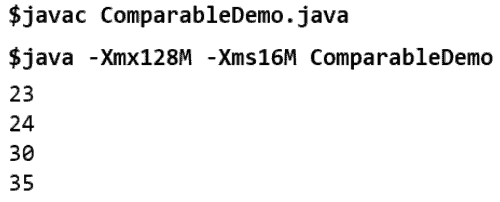## CompareTo() Method

The Java comparable interface consists only of a single method known as compareTo(). This method is used to compare the current object with other objects of similar type.

See the following syntax.

```public int compareTo (T obj)
```

See the following code.

```package com.tutorial.java.collections;

import java.util.ArrayList;
import java.util.Collections;

class Employee implements Comparable<Employee>
{ //implementing Comparable interface

private int e_id;
private String name;
private int age;

public Employee(int e_id, String name, int age)
{
this.e_id = e_id;
this.name = name;
this.age = age;
}

public int get_id()
{
return e_id;
}

public void set_id(int e_id) {
this.e_id = e_id;
}

public String getname() {
return name;
}

public void setName(String name)
{
this.name = name;
}

public int getAge()
{
return age;
}

public void setAge(int age)
{
this.age = age;
}

@Override
public int compareTo(Employee o)
{ // defining compareTo() method
return this.age - o.getAge();
}

}

public class JavaComparableExample
{  // defining driver class

static void details(Employee Employee)
{
System.out.println(Employee.get_id() + " - " + Employee.getname() + " - " + Employee.getAge());
}

public static void main(String[] args)
{
ArrayList<Employee> list = new ArrayList<Employee>();

System.out.println("Original List: ");
for (int i = 0; i < list.size(); i++)
{
details(list.get(i));
}

Collections.sort(list);

System.out.println("Sorted by age");
for (int i = 0; i < list.size(); i++)
{
details(list.get(i));
}
}

}```

See the following output.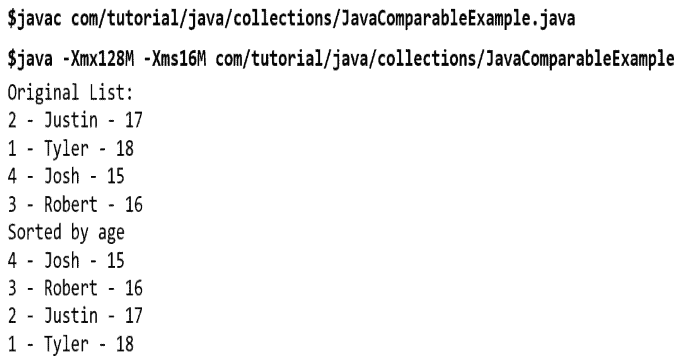## Working of compareTo() method

This method works by returning an integer value that could be negative positive or zero. It compares the current object by making a call to an object that is inside the method. Each condition means different results-

Negative: A negative number means that an object making the call is less than the object inside the method.

Positive: A positive number implies that the object making the call is more than the object that is in an argument.

Zero: It implies that the objects are equal.

The compareTo() method can be used to sort the following types of elements.

## Strings object

In Java, every time we create a string; in reality, an object is created. There are different ways to compare a string in java; one way is the compareTo() method.

See the following program.

```public class JavaStringCompare
{ // defining driver class

public static void main(String[] args)
{
String str = new String("ABC");// string object
System.out.println(str.compareTo("DEF")); //-3 (Integer.valueOf('A') - Integer.valueOf('D'))
System.out.println(str.compareTo("ABC")); //0 (equal string)
System.out.println(str.compareTo("abc")); //-32 (Integer.valueOf('A') - Integer.valueOf('a'))
System.out.println(str.compareTo("AB")); //1 (difference in length)
}
}```

See the following output.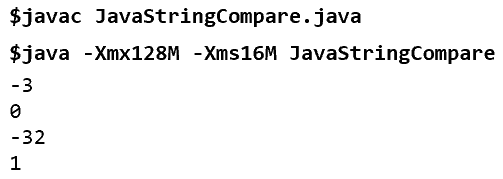## Wrapper class objects

In java sometimes, we have to use an object instead of primitive data types for software development purposes, so we need a Wrapper class to implement it.

See the following code example.

```public class WrapperClass {

public static void main(String args[]) {
Integer x = 9;

System.out.println(x.compareTo(7));
System.out.println(x.compareTo(9));
System.out.println(x.compareTo(11));
}
}```

See the output.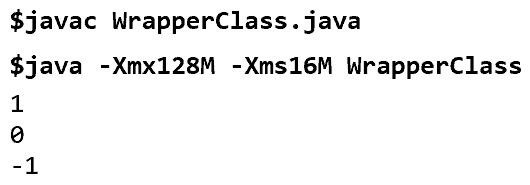## User-defined class object

The use of primitive data types is not always possible to work on a real-world related problem we have to use more complex real objects. These objects are knowns as user-defined class objects. See the following programming code.

```import java.util.*;

class Student implements Comparable<Student>
{  // implementing Comparabel interface
int rollno;
String name;
int age;
Student(int rollno,String name,int age)
{
this.rollno=rollno;
this.name=name;
this.age=age;
}

public int compareTo(Student st)
{
if(age==st.age)
return 0;
else if(age>st.age)
return 1;
else
return -1;
}
}
public class TestSort1
{ // defining driver class
public static void main(String args[])
{
ArrayList<Student> al=new ArrayList<Student>();

Collections.sort(al);
for(Student st:al)
{
System.out.println(st.rollno+" "+st.name+" "+st.age);
}
}
}```

See the following output.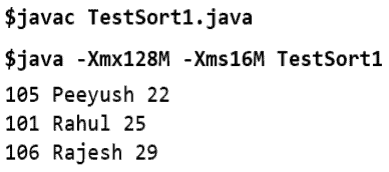That’s it for this tutorial.

This site uses Akismet to reduce spam. Learn how your comment data is processed.